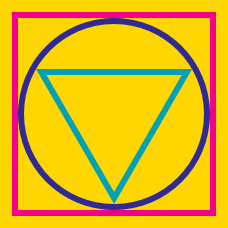Geometry

# Inscribed and Circumscribed Figures: Level 4 Challenges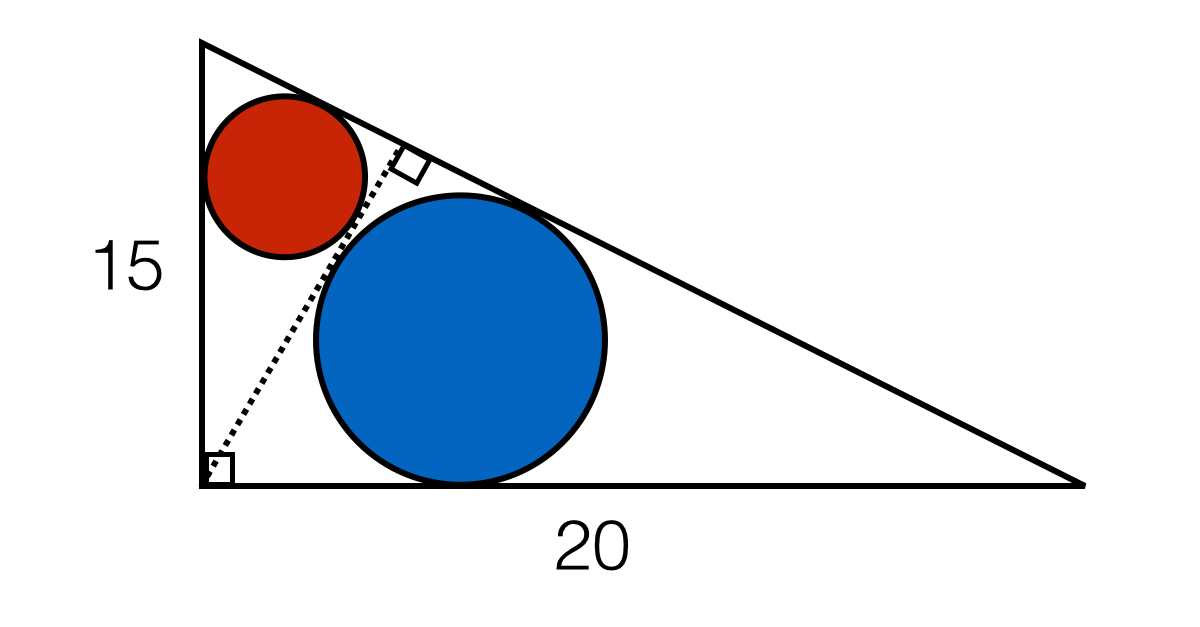In the right triangle above and altitude is drawn from the right angle to the hypotenuse. Circles are inscribed within each of the smaller triangles.

What is the distance between the centers of these circles?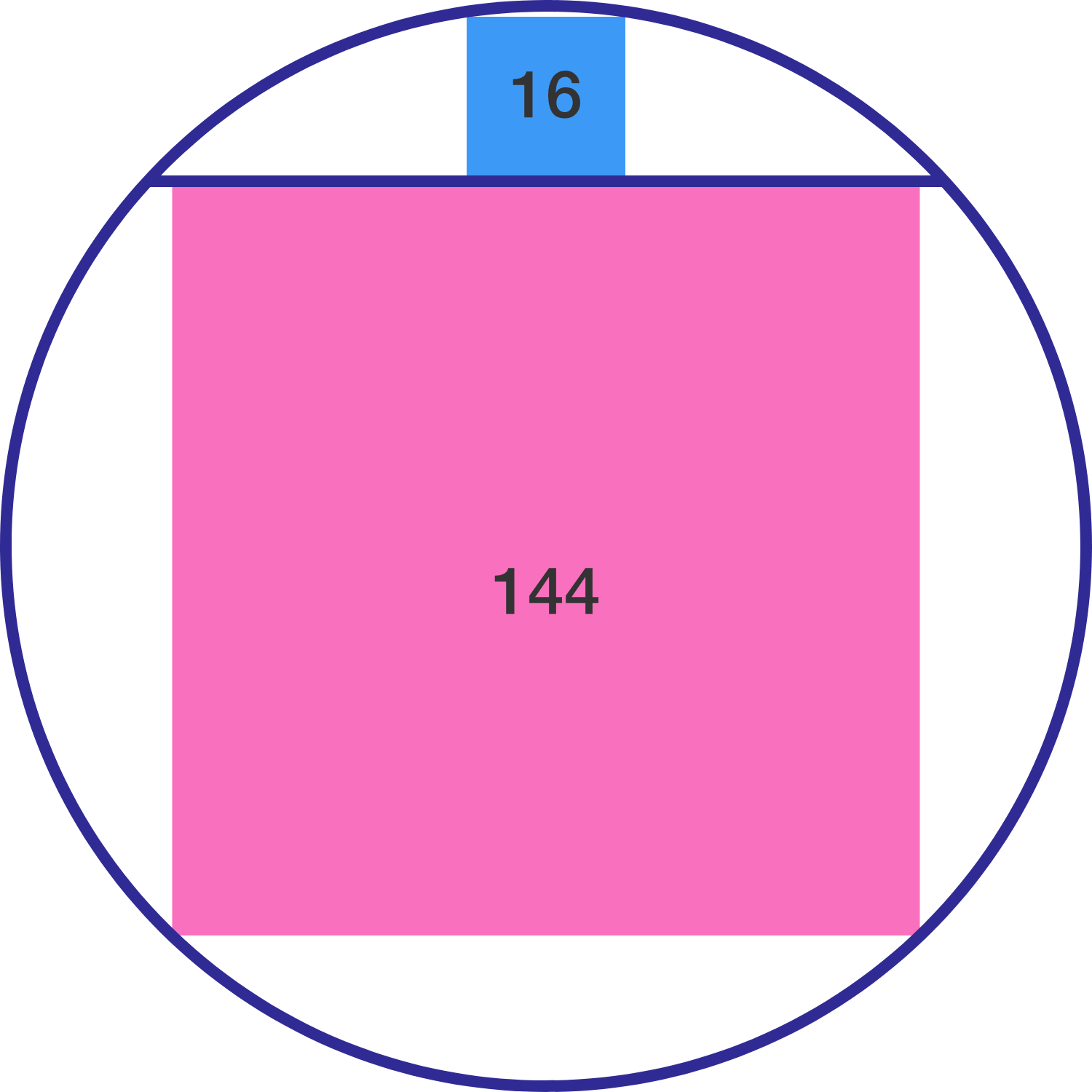A chord of a circle divides the circle into two parts such that the squares inscribed in the two parts have areas 16 and 144, respectively.

The radius of the circle is $\text{\_\_\_\_\_\_\_\_\_\_}.$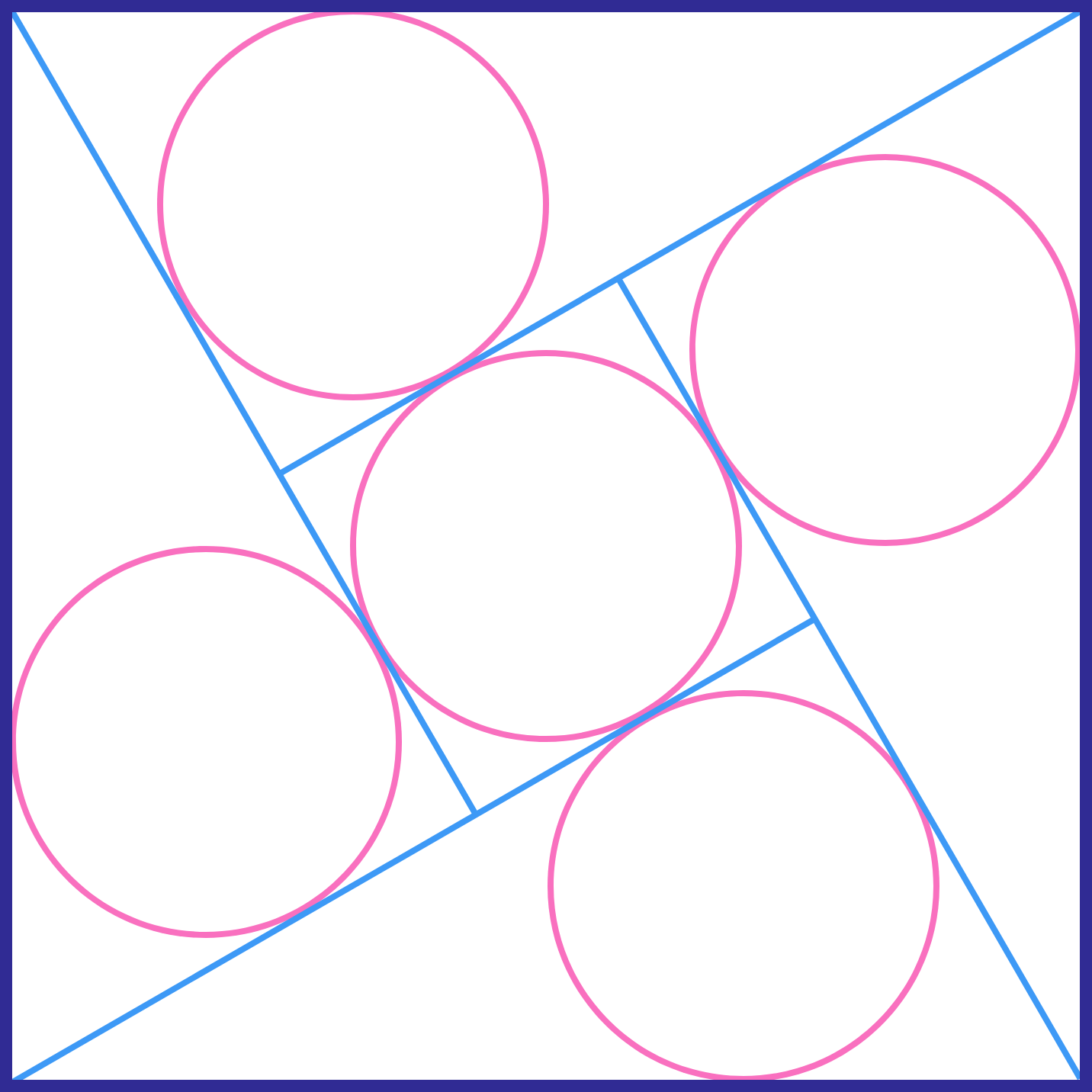The 5 circles have the same size. If the side of the large square is 1, what is the radius of each circle (to 3 decimal places)?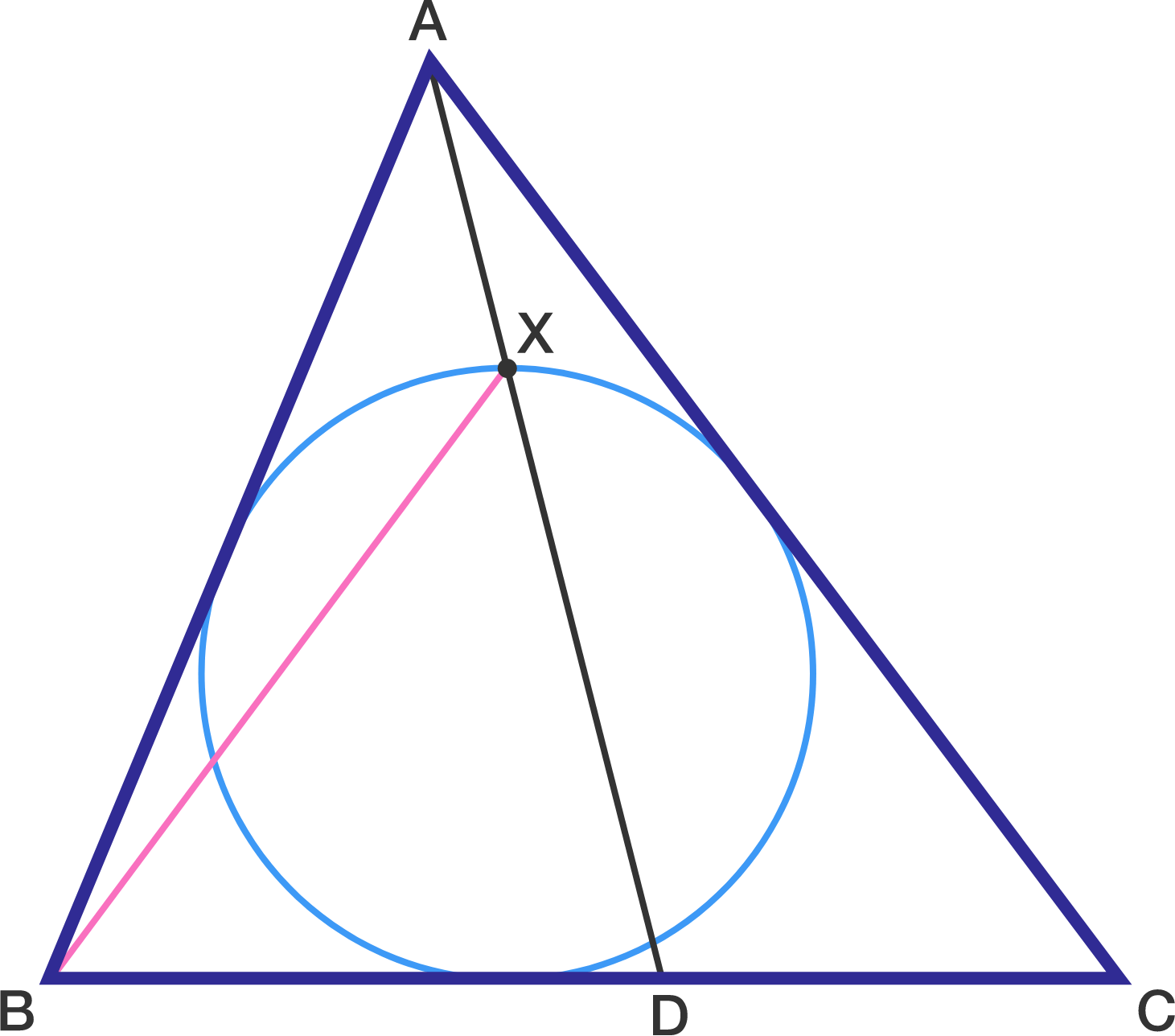Triangle $ABC$ is such that $AB = 13, BC = 14,$ and $CA = 15.$ A point $D$ on $BC$ is placed such that $AB + BD = AC + CD.$ Let $X$ be the intersection of $AD$ with the incircle of $ABC$ closest to $A.$ Find the length of $BX.$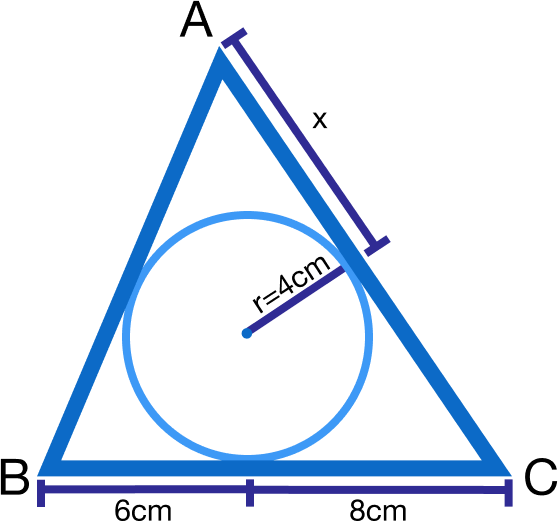Find the value of $x$.

×